# 量化交易 单因子有效性分析之 Alphalens

• 时间:
• 浏览:

### 单因子有效性分析之 Alphalens

• 概述
• Alphalens 的数据结构
• 参数
• factor 格式
• price 格式
• API
• 获取综合信息
• 因子 IC 分析
• 因子收益率系数分析

# 概述

Alphalens 是一个 Python 的第三方库, 专门用于选股因子的绩效分析.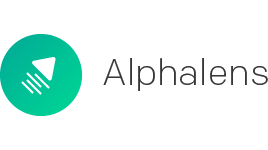``````pip install alphalens
``````

``````pip install git+https://github.com/quantopian/alphalens
``````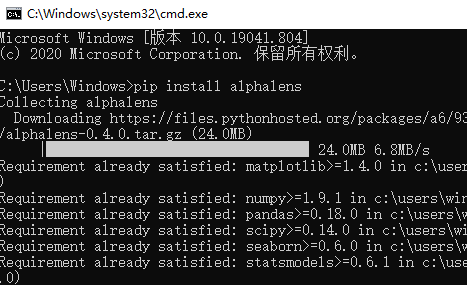# Alphalens 的数据结构

## 参数

``````alphalens.utils.get_clean_factor_and_forward_returns(factor, prices, groupby=None, quantiles=5, bins=None, periods=(1, 5, 10), filter_zscore=20, groupby_labels=None, by_group=bool, max_loss=0.35)
``````

factorpd.Series – MultiIndex一次一个因子数据，MultiIndex系列由时间戳（级别0）和资产（级别1）索引，包含单个alpha因子的值
pricepd.DataFrame所有股票的多天价格数据
groupbypd.Series - MultiIndex或dict行业分组信息， 保证每个股票都有行业分类
periods=(1, 5, 10)tuple默认计算了远期收益(预测能力)
groupby_labelsdict分组名称， 把所有的 行业贴上标签。比如说，(煤炭)中信对应数字 1，(钢铁)中信对应数字 2，以此类推。
max_loss=0.35float计算允许因子数据丢弃的最大百分比（0.00到1.00）,（例如NaN），未提供足够的价格数据来计算所有因子值的远期收益率，设置max_loss = 0避免数据出现缺失
by_groupbool分组计算可以理解成是传统的行业中性的做法
return: merged_data：pd.DataFrame - MultiIndex收益率、行业分组信息

### factor 格式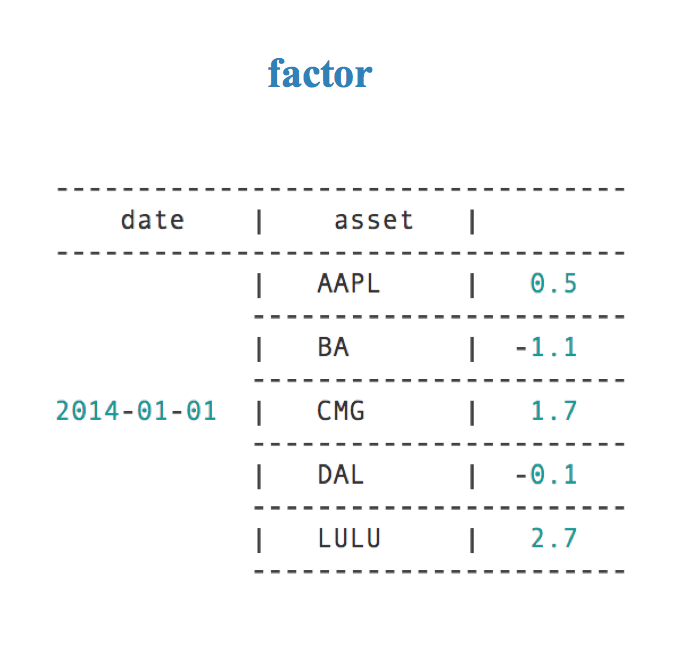### price 格式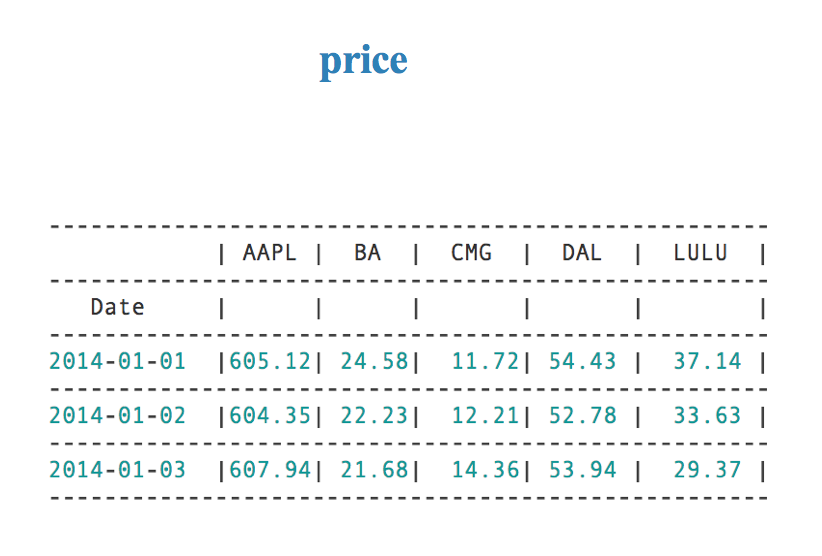# API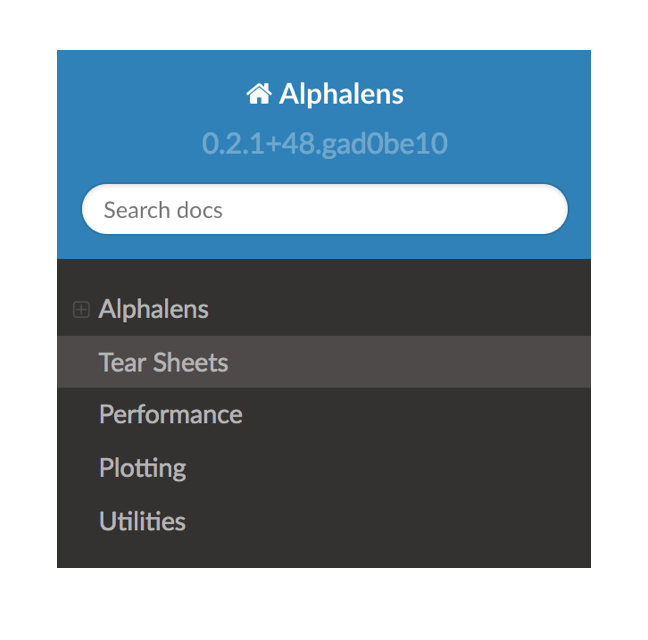``````from alphalens import performance
from alphalens import plotting
from alphalens import tears
from alphalens import utils
``````

## 获取综合信息

``````alphalens.tears.create_summary_tear_sheet(factor_data, long_short=True, group_neutral=False)
``````

## 因子 IC 分析

``````alphalens.performance.factor_information_coefficient(factor_data, group_adjust=False, by_group=False)
``````
• factor_data: 按日期 (级别 0) 和资产 (级别 1) 索引的 MultiIndex DataFrame, 包含单个 alpha 因子的值, 每个期间的正向收益
• by_group: 分组计算
• return: 返回 IC DateFrame

``````alphalens.tearse.factor_alpha_beta(factor_data, returns=None, demeaned=True, group_adjust=False, equal_weight=False)
``````

``````alphalens.tears.create_information_tear_sheet
``````

## 因子收益率系数分析

``````alphalens.tears.create_returns_tear_sheet(factor_data, long_short=True,group_neutral=False, by_group=False)
``````